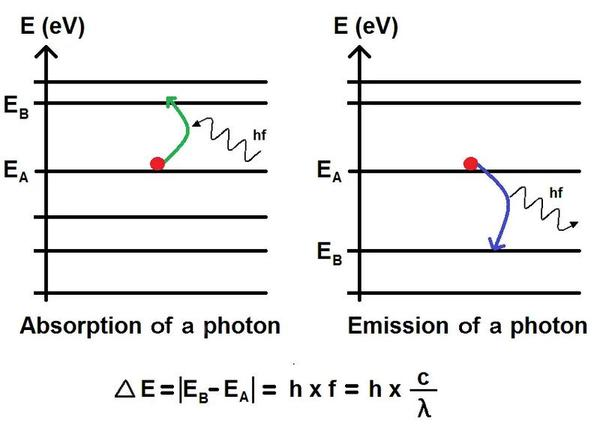# Planck's equation

## E = hfAutomatic translation Updated April 03, 2023

The Planck equation (1900), named after the German physicist Max Planck (1858-1947), is a formula that describes the relationship between the energy of a photon and the frequency of the corresponding light.
This equation was developed by the physicist to explain the spectral distribution of black body radiation which was an enigma at the time.
Indeed, when one calculated the spectral density of the energy radiated by a black body at a given temperature with the classical theory of electromagnetism, one did not obtain the real distribution of the energy observed for the black bodies.
The classical theory of electromagnetism assumed that energy was continuous, that is, electromagnetic waves could have any energy.
To solve this enigma, Planck hypothesizes that energy is not exchanged continuously but in small discrete "packets" which he calls "quanta". This assumption meant that the electromagnetic energy could not take any value, but only multiples of a certain basic energy equal to the frequency multiplied by a constant.
This equation is today one of the cornerstones of quantum physics and is expressed in the following form:
E = hf where E represents the energy of the photon, f the frequency of light and h Planck's constant which has a value of 6.626 x 10^-34 joule-second.
This means that the energy E of an electromagnetic photon is directly proportional to its frequency ν, and that the proportionality constant is Planck's constant h. This relationship is known today as the Planck-Einstein equation.

What is E=hf for?
This equation had important implications for understanding the nature of light, which at the time was considered an electromagnetic wave only.
Planck's equation led to the development of quantum mechanics, which is the branch of physics that studies the properties and behavior of subatomic particles.
Planck's constant h, which relates the energy of photons to their frequency, is a fundamental constant of quantum physics because it is used to quantify the energy associated with electromagnetic interactions and all quantum processes.
It plays an important role in the understanding of many phenomena such as wave-particle duality, emission and absorption of photons, quantum transition energies in atoms and molecules, electrical conductivity of solids, production of electromagnetic radiation in particle accelerators, understanding star formation, etc.
It is used in many technological fields, especially for the study of spectroscopy, the design of semiconductors, the production of lasers, the design of solar cells, etc.
Planck's constant is found in many equations of quantum physics, notably in the Schrödinger equation, which describes the time evolution of the quantum states of a system. But also in the Heisenberg uncertainty relation, which states that there is a fundamental limit to the precision with which it is possible to simultaneously measure the position and the momentum of a particle.### Image: E=hf ⇒ E=h c/λ ⇒ λ=hc/E h = 6.626 x 10^-34 joule-second

NB: joules-seconds (Js) are units of action and angular momentum which represent masses (in kg) multiplied by a speed (in m/s) multiplied by a position.

1997 © Astronoo.com − Astronomy, Astrophysics, Evolution and Ecology.
"The data available on this site may be used provided that the source is duly acknowledged."